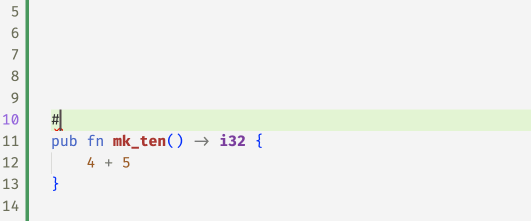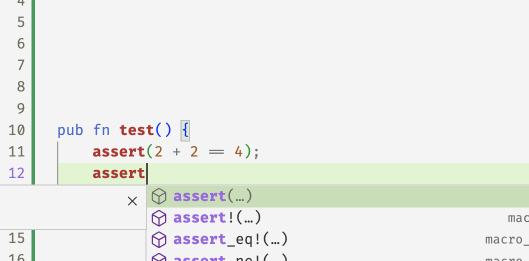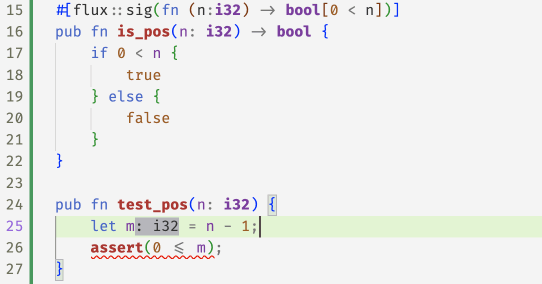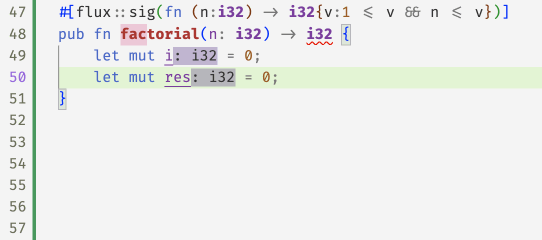# Introducing Flux

Online demo

Types bring order to code. For example, if a variable `i:usize` then we know `i` is a number that can be used to index a vector. Similarly, if `v:vec<&str>` then we can be sure that `v` is a collection of strings which may be indexed but of course, not used as an index. However, by itself `usize` doesn't tell us how big or small the number and hence the programmer must still rely on their own wits, a lot of tests, and a dash of optimism, to ensure that all the different bits fit properly at run-time.

Refinements are a promising new way to extend type checkers with logical constraints that specify additional correctness requirements that can be verified by the compiler, thereby entirely eliminating various classes of run-time problems.

We're excited to introduce Flux, a refinement type checker plugin that brings this technology to `Rust`.

## Indexed Types

The most basic form of refinement type in `flux` is a type that is indexed by a logical value. For example

TypeMeaning
`i32`The (singleton) set of `i32` values equal to `10`
`bool[true]`The (singleton) set of `bool` values equal to `true`

### Post-Conditions

We can already start using these indexed types to start writing (and checking) code. For example we can write the following specification which says that the value returned by `mk_ten` must in fact be `10`

``````#![allow(unused)]
fn main() {
#[flux::sig(fn() -> i32)]
pub fn mk_ten() -> i32 {
5 + 4
}
}``````

but when you compile it, `flux` will say

``````error[FLUX]: postcondition might not hold
--> src/basics.rs:7:5
|
7 |     5 + 4
|     ^^^^^
``````

The error says that that the postcondition might not hold which means that the output produced by `mk_ten` may not in fact be an `i32` as indeed, in this case, the result is `9`! You can eliminate the error by replacing the body with `5 + 5` or just `10`.### Pre-Conditions

Here's a second example that shows how you can use an index to restrict the space of inputs that a function expects.

``````#![allow(unused)]
fn main() {
#[flux::sig(fn (b:bool[true]))]
pub fn assert(b:bool) {
if !b { panic!("assertion failed") }
}
}``````

Here, the refined specification for `assert` says that you can only call it with `true` as the input. So if you write

``````#![allow(unused)]
fn main() {
fn test(){
assert(2 + 2 == 4);
assert(2 + 2 == 5); // fails to type check
}
}``````

then `flux` will complain that

``````#![allow(unused)]
fn main() {
error[FLUX]: precondition might not hold
--> src/basics.rs:12:5
|
12 |     assert(2 + 2 == 5); // fails to type check
|     ^^^^^^^^^^^^^^^^^^
}``````

meaning that the call to `assert` fails to establish that the input is indeed `true` (as of course, in this case, it is not!)## Index Parameters and Expressions

Its not terribly exciting to only talk about fixed values like `10` or `true`. To be more useful, `flux` lets you index types by refinement parameters. For example you can write

``````#![allow(unused)]
fn main() {
#[flux::sig(fn(n:i32) -> bool[0 < n])]
pub fn is_pos(n: i32) -> bool {
if 0 < n {
true
} else {
false
}
}
}``````

Here, the type says that `is_pos`

• takes as input some `i32` indexed by `n`
• returns as output the `bool` indexed by `0 < n`

in other words, the output is `true` exactly when `0 < n`.

We might use this function to check that:

``````#![allow(unused)]
fn main() {
pub fn test_pos(n: i32) {
let m = if is_pos(n) { n - 1 } else { 0 };
assert(0 <= m);
}
}``````## Existential Types

Often we don't care about the exact value of a thing -- but just care about some properties that it may have. For example, we don't care that an `i32` is equal to `5` or `10` or `n` but that it is non-negative.

TypeMeaning
`i32{v: 0 < v}`The set of `i32` values that positive
`i32{v: n <= v}`The set of `i32` values greater than or equal to `n`

Flux allows such specifications by pairing plain Rust types with assertions 1 that constrain the value. For example, we can rewrite `mk_10` with the output type `i32{v:0<v}` that specifies a weaker property: the value returned by `mk_ten` is positive.

``````#![allow(unused)]
fn main() {
#[flux::sig(fn() -> i32{v: 0 < v})]
pub fn mk_ten() -> i32 {
5 + 5
}
}``````

Similarly, you might specify that a function that computes the absolute value of an `i32` with a type which says the result is non-negative and exceeds the input `n`.

``````#![allow(unused)]
fn main() {
#[flux::sig(fn (n:i32) -> i32{v:0<=v && n<=v})]
pub fn abs(n: i32) -> i32 {
if 0 <= n {
n
} else {
0 - n
}
}
}``````

As a last example, you might write a function to compute the factorial of `n`

``````#![allow(unused)]
fn main() {
#[flux::sig(fn (n:i32) -> i32{v:1<=v && n<=v})]
pub fn factorial(n: i32) -> i32 {
let mut i = 0;
let mut res = 1;
while i < n {
i += 1;
res = res * i;
}
res
}
}``````

Here the specification says the input must be non-negative, and the output is at least as large as the input. Note, that unlike the previous examples, here we're actually changing the values of `i` and `res`.Can you guess why the copilot suggestions failed to pass flux, and what refinements were inferred for `i` and `res` in the fixed code at the end?

## Summary

In this post, we saw how Flux lets you

1. decorate basic Rust types like `i32` and `bool` with indices and constraints that let you respectively refine the sets of values that inhabit that type, and

2. specify contracts on functions that state pre-conditions on the sets of legal inputs that they accept, and post-conditions that describe the outputs that they produce.

The whole point of Rust, of course, is to allow for efficient imperative sharing and updates, without sacrificing thread- or memory-safety. Next time, we'll see how Flux melds refinements and Rust's ownership to make refinements happily coexist with imperative code.

1

These are not arbitrary Rust expressions but a subset of expressions from logics that can be efficiently decided by SMT Solvers